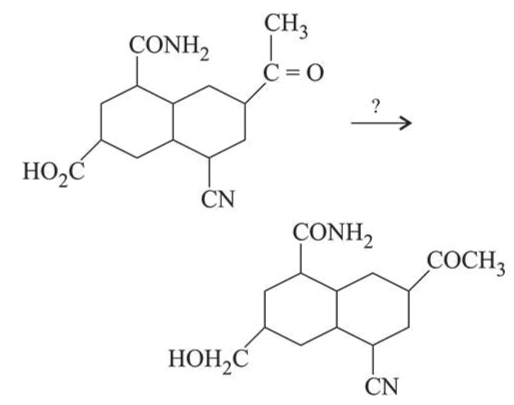# The most suitable reagent for the given conversion is:Question:

The most suitable reagent for the given conversion is:1. $\mathrm{B}_{2} \mathrm{H}_{6}$

2. $\mathrm{NaBH}_{4}$

3. $\mathrm{LiAlH}_{4}$

4. $\mathrm{H}_{2} / \mathrm{Pd}$

Correct Option: 1

Solution:

$\mathrm{B}_{2} \mathrm{H}_{6}$ is a very selective reducing agent and usually used to reduce acid to alcohol.